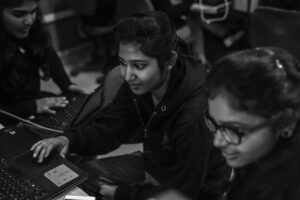Back“We want to create a robot with a brain”

In today’s techno world a man wants to make his/her life as smooth as possible and day by day we are getting dependent on electronic gadgets. To give the computers the brain like a human, for that we need to give computers the brain like structure made up of the nervous system like a human.

Every neuron is working in the human body for giving reactions and mood swings. So the robot developed by scientist needs a lot of improvement in these robots because they are machines and can’t show their emotions like a human. So the subject deals with both the fragments human and machines. First, we need to understand the psychology of human and then implement the same in machines(robot).

In a neural network, we cover both the BNN and ANN with its learning, the programs which are helpful for understanding the neurons. These programs are done on MATLAB.

MATLAB (matrix laboratory):

It is a numerical computing environment. MATLAB allows matrix manipulations, plotting of functions and data, implementation of algorithms.

In B. S. Anangpuria Institute of Technology &amp; Management at our department of Computer Science Engineering, we start our student with basic of MATLAB functions where students get the awareness of matrix multiplication, transpose of the matrix and many other functions that are required for their project.

The NEURAL NETWORK lab comprises of a couple of Practical’s which provide the students with all the basic and essential skills. To understand the research work of AI that helps us to develop new applications in the field of artificial intelligence “A man wants to create a robot having the same functionality like a human that could also have felt like a human” in short intelligence.

The First Part that we start with proving the truth table of AND, OR, NAND gates which are the topics under linearly separable i.e. a single line can separate out the different patterns, shapes, and images. But there are certain issues that need to be sorted out like a linearly inseparable problem that we can do in Matlab using XOR gate.

The Second part contains the information about the energy that is required to transfer the information from one neuron to another neuron .but in ANN this energy is considered as the activation function. In Matlab student can write a program and can view the different graphs that are created using different activation function such as LTF(linear transfer function), Bipolar, sigmoidal, hardlim etc.

These Practical’s provide Computer Science &amp; Engineering Students the real-time knowledge of how the researchers are working on these projects.

To develop any application it’s the foremost requirement that the expected requirements/results are equal to the obtained result. As a human, we can find the problems and their solutions but a machine requires some parameters to count the error.

Therefore,

Error = ½(expected output-obtained output)/2

If there is no error in the network then it is considered to be the best network but if there is some error then we need to backtrack the network.so we apply the backpropagation algorithm on it.

The Thirds Part of this lab includes the other factor of human i.e the memory and in the same way, we try to give memory to our ANN .in this phase we provide the student with the knowledge about two types of memory auto-associative and hetero-associative memory. The student implements their coding on Matlab for checking the memory of the machine.

In the fourth part, our students perform their work on NN tool. In NN tool student work on simulator where he can visualize the proper iterations of network side by side they can find the errors and can also remove the error by changing the data values. Student gets the exposure of real-time environment.

At the end of semester students perform one project on Matlab where the student gets the full exposure to the new era of software world and technology.

We at Department of Computer Science &amp; Engineering make sure that our student attending these Labs not only get equipped with all necessary information but also able to work with these research-oriented software’s.